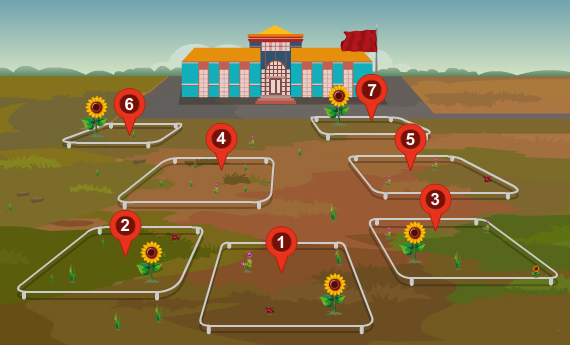Study of plant population frequency by quadrat method

# Aim

Our aim is to study plant population frequency by quadrat method.

# Theory

In ecology it is useful to know the frequency of certain plant species in a certain place, or at a certain time. Frequency can be defined as the degree of uniformity of the occurrence of individuals of a species within a plant community.  However, finding the frequency of plant species is very difficult for a large populations or extensive habitats. A widely used method for plant frequency sampling is by quadrat method.

Scientists usually calculate the plant population frequency using the quadrat method. A quadrat is a sample plot of a specific size used for the study of population or a community. Quadrats are used in many different scientific disciplines like vegetation assessment, including plant density, plant frequency and plant biomass. Frequency is highly influenced by the size and shape of the quadrats used. The area that is chosen for study must not be so big that it cannot be sampled adequately, or so small that the habitat is difficult for sampling. For herbaceous vegetation a metre square quadrat is normally used.

## Frequency

Determining plant population frequency using quadrat method has become popular primarily because it is relatively simple and objective. Frequency indicates the number of times a plant species is present within a given number of sample quadrats. It is measured by noting the presence of a species in random sample areas which are distributed as widely as possible throughout the area of study. Once analyzed, the sample data enables the scientist to calculate population frequency of the entire population by the following method:
%Frequency=(Number of sampling units in which the species occurs)/(Total number of sampling units employed for the study)*100

## How to calculate population frequency?

The image below depicts a plot which is divided into seven quadrats.Observe the presence sunflower plant in each quadrat.  In this case, sunflowers are present in five quadrats among the seven.

Hence, the percentage frequency of sunflower is:

%Frequency=5/7*100=71.42

## Variations in Plant Distribution

Variation in distribution is caused by several factors like soil conditions, vegetative propagation, quantity and dispersal of seeds, grazing or other biotic activities and predation by insects or diseases. Some species abundantly spread all over the area have a chance of occurring in all the sampling quadrats and therefore, its frequency will be 100. The plants with high frequency are wide in distribution.

# Learning Outcomes:

• Students understand the term quadrate method.
• Students understand how to calculate plant population frequency.
• Students do the experiment better in the real lab having gone through the animation and simulation.

Cite this Simulator: